# MIRR Guide

A financial term used to explore and evaluate the worth and profitability of a possible investment or project.Reviewed by

Patrick Curtis

Expertise: Private Equity | Investment Banking

Updated:

January 4, 2023

The MIRR, or Modified Internal Rate of Return, is a financial term used to explore and evaluate the worth and profitability of a possible investment or project.It enables businesses and investors to pick the most profitable project or investment based on predicted returns. It's just a variation of the Internal Rate of Return (IRR).

The modified version assists companies in capital planning by comparing the feasibility or return of one investment or project to that of others.

In contrast to IRR, which exaggerates the attractiveness of any investment while misleading investors about predicted greater returns, MIRR provides a fair estimate of the ROI investors may expect.

In MIRR, positive cash flows will be reinvested at the cost of capital, while new expenditures will be funded at the expense of financing. At the same time, the traditional IRR presupposes that project cash flows will be reinvested at the IRR.

As a result, the Modified Internal Rate of Return more precisely depicts a project's cost and profitability.

Furthermore, MIRR only provides one solution for each cash flow sequence, but IRR may supply two (negative and positive). The Modified Internal Rate of Return is intended to yield a single solution, avoiding the need for several IRRs.

## What does MIRR tell you?

It assesses various investments or projects that a business or investor may undertake.If it exceeds the predicted return, the investment should be made. Conversely, the project should be rejected if it is less than the estimated return. In addition, if two projects are incompatible, the one with the higher Modified Internal Rate of Return should be undertaken.

It enables project managers to adjust the expected rate of reinvested growth from one stage to the next. While any precise anticipated reinvestment rate can be used, the most typical way is to provide the average predicted cost of capital.

Using assessment criteria, it presents the ROI in a better, more precise, and realistic manner. It computes the investment cost (capital) and the interest generated on money to be reinvested.

## NOTE

Organizations do computations to estimate revenues, earnings, or expenditures.

The Modified Internal Rate of Return calculator generates more accurate returns, allowing managers to regulate the predicted reinvestment rate from future cash flows more precisely.

The standard IRR exaggerates investment returns, providing investors with false optimism. MIRR, on the other hand, evaluates various expenses to determine the returns on investment. Consequently, it helps minimize capital planning mistakes and unrealistic expectations.

As a result, it protects investors and business owners/managers from being deceived. But, aside from that, it can assist with various other financial aspects:

• Scores investments of comparable size according to the opportunities they bring.
• Suggests an attractive investment or project when it exceeds anticipated return and vice versa
• Changes the IRR of an investment or project and computes the change between the reinvestment rate and the investment return.
• Removes any difficulties caused by numerous IRRs at the same time.
• Allows for adjustments to the projected growth rate of reinvestments at various phases of project completion.
• It allows you to enter any reinvestment rate and base your calculations on that.Before we go into its calculations and formulas, let's look at the three methodologies employed:

1. Discounted Approach

All negative cash flows are added to the starting cost and discounted to the present investment.

2. Reinvestment Approach

All positive and negative cash flows (except for the first) are compounded through the end of the project's lifetime, and the IRR is computed on the total.

3. Combination Approach

It is a hybrid strategy that combines and employs the two prior strategies. To calculate the IRR, all negative cash flows are discounted to the current investment, while all positive cash flows are compounded.

## MIRR Formula and Calculation

There are two approaches for calculating the MIRR: the two-stage approach and the straight formula method. Your demands and the tools and resources available to you will decide the strategy you select.The two-stage technique involves calculating the IRR of the investment or project and then using that rate to discount the cash flows to their present value. The Modified Internal Rate of Return is determined by dividing the present value by the investment cost.

The formula approach entails employing a formula to calculate the Modified Internal Rate of Return.

To calculate the Modified Internal Rate of Return formula for a project, we must first compute the future value of a firm's positive cash flows discounted at the firm's cost of capital and the present value of a firm's negative cash flows discounted at the firm's cost of capital.Firms and investors utilize the Modified Internal Rate of Return formula in capital budgeting as follows: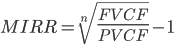Where,

• FVCF = After discounting the reinvestment rate or cost of capital, the future cost of positive cash flows is as follows: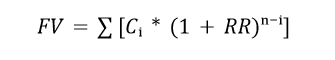Ci denotes positive cash flow, while RR is the reinvestment rate.

• PVCF = The current value of the negative cash flows after subtracting the firm's finance cost: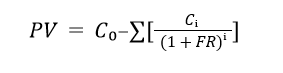C0 represents the negative cash flow, while FR represents the financing rate.

• n is the number of years

Manually calculating the Modified Internal Rate of Return using the formula might be tedious and error-prone. As a result, financial businesses and stock exchanges employ spreadsheet tools such as Microsoft Excel to calculate the return on investment.The Excel function is as follows:

=MIRR(values, finance_rate, reinvest_rate)

Where:

• Values represent the individual cash flows from each period in the series.
• finance_rate is the cost of borrowing or interest expense in the case of negative cash flows
• reinvest_rate is the compounding rate of return at which positive cash flow is reinvested

## NOTE

For the formula to operate properly, the initial expenditure must be entered as a negative amount in Excel.

## The Difference Between MIRR and IRR

Despite its popularity among corporate leaders, the internal rate of return (IRR) metric overestimates projected profitability and can lead to capital planning mistakes based on an overly optimistic expectation.This deficiency is compensated for by the modified internal rate of return, which gives managers greater control over the predicted reinvestment rate from future cash flow.

A calculated IRR is akin to an inverted compounded growth rate. It must account for the return on investment and the reinvested cash flows.

The IRR does not accurately represent how cash flows are reinvested in future projects. The issue is that it implies that future positive cash flows will be reinvested instead of project or business capital (i.e., the required rate of return).

Rather than at the rate of creation, cash flows are usually reinvested at the cost of capital. IRR assumes a consistent rate of growth across operations. Using basic IRR estimations, it is quite easy to overstate potential future value.

Another significant difficulty with IRR arises when a project has positive and negative cash flow phases. In some circumstances, the IRR produces a significant value, leading to doubt and misunderstanding. The Modified Internal Rate of Return also handles this.

One thing is certain: it provides improved control and precision as it always provides a single answer compared to the uncertainty or confusion caused by the two answers provided by IRR.

## NOTE

In practice, the MIRR Excel function is regarded as a more cautious statistic than the IRR function (and usually results in a lower return).

The standard IRR function is commonly used in financial modeling, particularly in private equity and investment banking. This is because transactions are evaluated in isolation rather than with the impact of another investment assumption stacked on top.

The Modified Internal Rate of Return requires an additional assumption, which may make two unique transactions appear less similar.

IRR is akin to inverted compounding of the growth rate, but the modified version helps managers anticipate a realistic return and plan their objectives appropriately.

The issue with the modified version is that it needs you to compute a cost of capital estimate to make a choice, which can be subjective and vary based on the assumptions used.When weighing many investment possibilities at once, the Modified Internal Rate of Return, like the IRR, may present information that leads to suboptimal judgments that do not maximize value. It does not compute the absolute effects of different investments.

MIRR is also a difficult concept to grasp for those without a financial background. There is a discussion within academic circles over the theoretical basis for the Modified Internal Rate of Return calculation.

Furthermore, because it is not as extensively utilized as traditional IRR, it will need more socialization, buy-in, and explanation from most businesses, banks, accounting firms, and institutions.

In contrast, MIRR is frequently regarded to be more accurate than IRR and other equivalent estimates.

Here is a table that summarizes the main difference:

Difference
BasisMIRRIRR
CalculationUses cost of capital as a discount rateUses projected cash flows
AssumptionsCash flows reinvested at the cost of capitalCash flows reinvested at IRR
Handling of non-normal cash flowsProduces single solution for non-normal cash flowsCan produce multiple solutions or no solution
AccuracyHighly accurate Provides a precise estimate of the returnsOverstates the projected return on investment
SuitabilitySuitable for variable or non-normal cash flowsSuitable for stable cash flows

## An Excel Example of the Modified Internal Rate of Return

The example below shows how the Modified Internal Rate of Return varies from normal IRR in the most obvious way.In the following example, we consider two distinct projects. The overall amount of cash received during the life of the investment is the same in both circumstances; the only variation is the timing of those cash flows.

Assuming that:

• The initial investment is the same in both projects and equals $5,000 • Major positive cash flow is$9,000, and the same in both cases
• Major cash flows are timed for the last year in Project 1 and the first year for project 2
• The reinvestment rate is 0% for MIRR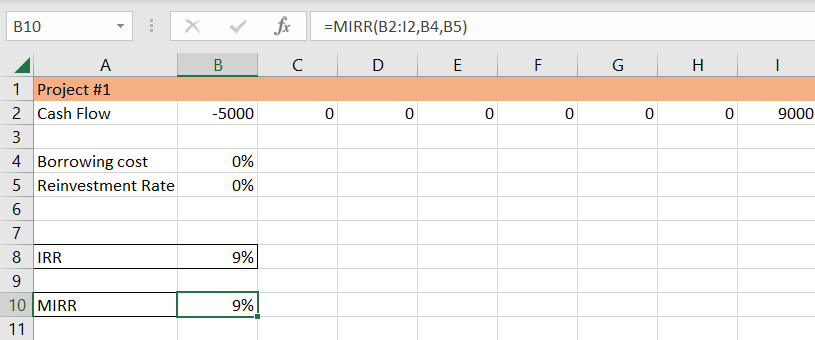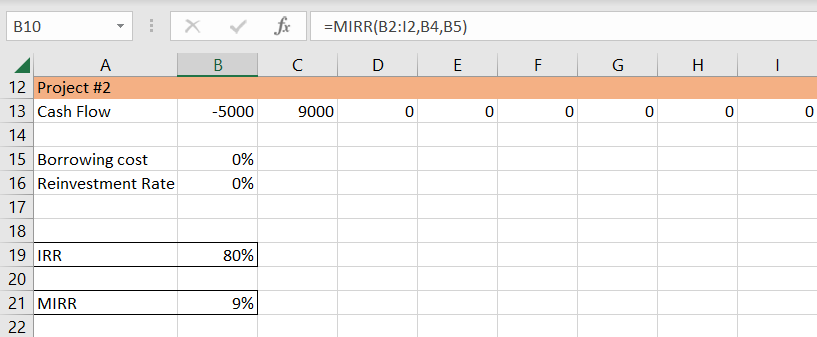The return computed by MIRR and IRR in Project #2 differs significantly, as shown in the graphic above. There is no difference in Project #1.

Let's look at the reasons why.

Project #1

Because all cash flow is collected at the end of Project #1, the reinvestment rate is immaterial.

It is critical to demonstrate this instance to demonstrate that reinvestment is irrelevant when a project has only one ultimate cash flow.

A zero-coupon bond or a leveraged buyout (LBO) are two examples of cash flow used to service debt until the firm is sold for a huge lump sum.

Project #2

In Project #2, however, almost all of the cash flow is received in year one. This indicates that the reinvestment rate will significantly impact the project's total IRR.

We can use an extreme example to demonstrate the concept since we set the reinvestment rate to 0%.

Remember that IRR is the discount rate that reduces an investment's Net Present Value (NPV) to zero. So, the IRR example simply states that the $9,000 cash flow in year one must be discounted by 80% to arrive at an NPV of$0.

## Key Takeaways

• MIRR is a financial measure used to assess the feasibility of a new investment or project.
• Based on the assessment factors it evaluates, it gives companies and investors a clearer, better, and more accurate image of the ROI. It is computed by combining the investment cost with the income earned on the money to be reinvested.
• The IRR is computed by deducting the growth from the initial investment. As a result, it relies on the inverted compounding of the growth rate. MIRR, on the other hand, allows firms to compute returns based on expected current reinvestment rates at each step.
• The modified internal rate of return gives an accurate and reliable estimate of the ROI that investors might expect.### Everything You Need To Master Excel Modeling

To Help You Thrive in the Most Prestigious Jobs on Wall Street.

Researched and authored by Wissam El Maouch | LinkedIn

Reviewed & Edited by Ankit SinhaLinkedIn